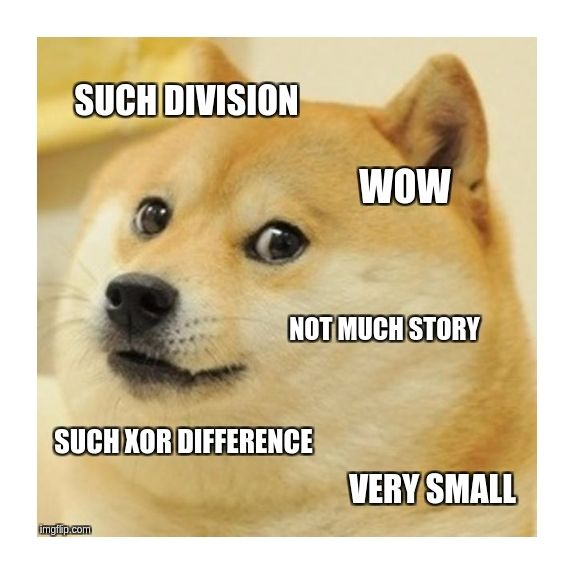시간 제한 메모리 제한 제출 정답 맞은 사람 정답 비율
6 초 512 MB 31 9 9 30.000%

## 문제

Every good problemset needs a problem that can be summarized in a single doge meme. Today's problemset is no different.Given a multiset $S$ of non-negative integers, divide it into two multisets $A$ and $B$ in a way that minimizes $|\text{xor}(A) - \text{xor}(B)|$. Here $\text{xor}(X)$ denotes the bitwise XOR of all elements of $X$.

Note that one of the multisets $A$ and $B$ can be empty, and XOR of an empty multiset is $0$.

It is enough to output the minimum possible value of $|\text{xor}(A) - \text{xor}(B)|$.

## 입력

The first line of input contains the number of test cases $z$ ($1 \leq z \leq 50$). The descriptions of the test cases follow, two lines per test case.

The first line of every test case contains an integer $n$ ($1 \leq n \leq 10^5$) -- the size of the multiset.

The second line contains $n$ integers $x_i$ ($0 \leq x_i \leq 10^{18}$) -- elements of the multiset.

## 출력

For each test case output one integer: the smallest possible difference of XORs.

## 예제 입력 1

2
4
1 2 3 4
5
3 7 3 9 5


## 예제 출력 1

4
5